# How to Sum Values Based on Selection of Drop-Down List in Excel?

We can do SUM for a list or a range of values easily if we select all values together and then apply SUM function in excel. But if we only want to sum part of them based on the selection in a dropdown list, how can we do? For example, suppose we have two lists, one list records some fruits, and they can be duplicate in this list, the other list records the amount, if we create a filter on fruits and just pick one of them, how can we sum the total amount of this filtered fruit. Though we can calculate the total amount one cell by another cell, this is not very simple. So, in this free tutorial, we will introduce you a simple way (through a formula) to sum values based on the selection of dropdown list.

Precondition:

Prepare a table contains two columns. The first column records some fruits, they may be duplicate. The second column records the ‘Amount’ for fruits. We want to create a dropdown list for fruit, and after selecting any fruit from dropdown list, its total amount is calculated automatically and just displayed next to the selection.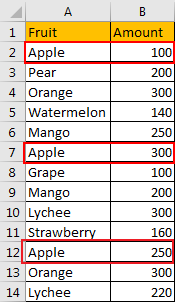## Method: Sum Values Based on Selection Of Drop-Down List

Step 1: Copy ‘Fruit’ column to another column first. For example, column D.

Step 2: On column D, click Data in ribbon, then click Remove Duplicate under Data Tools group to remove duplicate fruits from new fruit list.Step 3: On Remove Duplicates Warning dialog, select the second option ‘Continue with the current selection’. Then click Remove Duplicates. This step makes sure you only remove the duplicate values from your new fruit list and keep the original fruit list unchanged.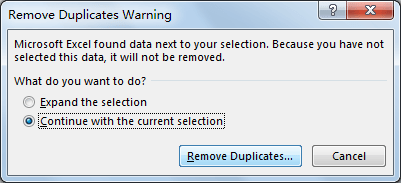Step 4:Remove Duplicates’ dialog pops up. Just click OK.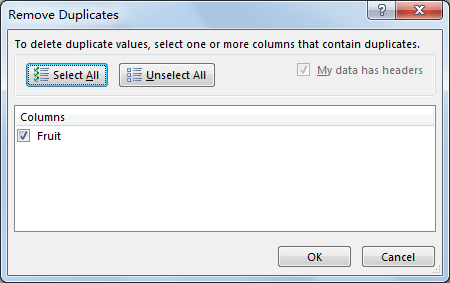The you will notice that duplicate values are removed from column D. All fruits are unique.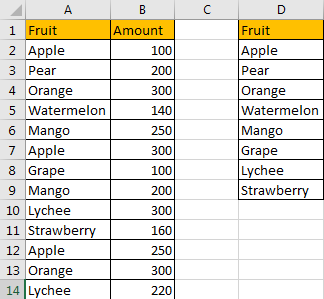Step 5: Add two new columns. See screenshot below.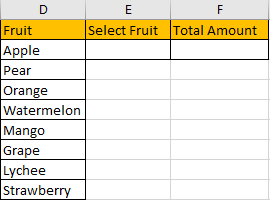Step 6: Select on E2, then click Data->Data Validation under Data Tools group.Step 7: On Data Validation window. There are three tabs. Click the first ‘Settings’, select ‘List’ in Allow dropdown list; select =\$D\$2:\$D\$9 (new fruit list) in Source textbox.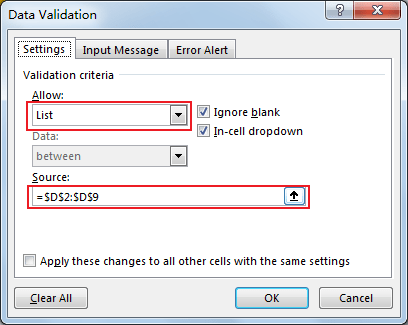Step 8: If you want to get some prompt message, you can click the second tab ‘Input Message’, and enter title and message. Then click OK.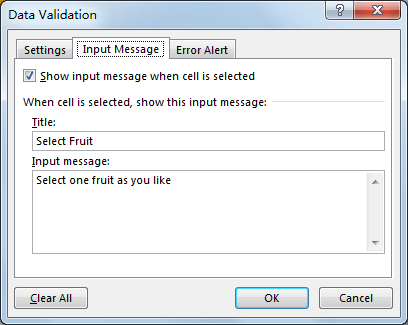Step 9: Click on E2, verify that ‘Select Fruit’ message pops up.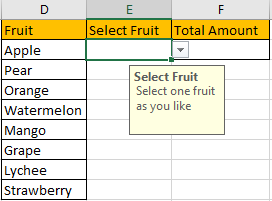Step 10: Select a fruit in dropdown list, for example ‘Orange’.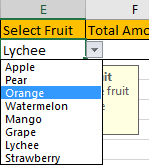Step 11: In F2, enter the formula =SUMPRODUCT(SUMIF(\$A\$2:\$A\$14,\$E\$2,\$B\$2:\$B\$14)).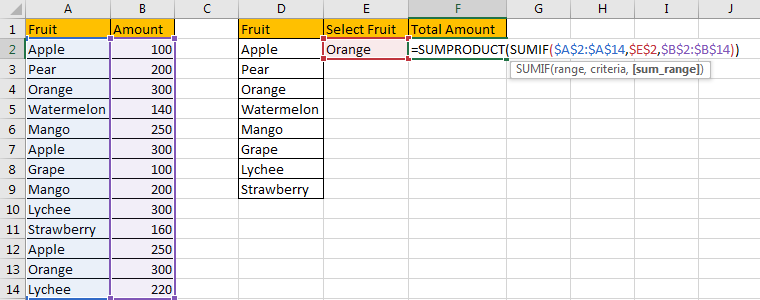Step 12: Press Enter and check the result. Verify that total amount for orange is calculated correctly.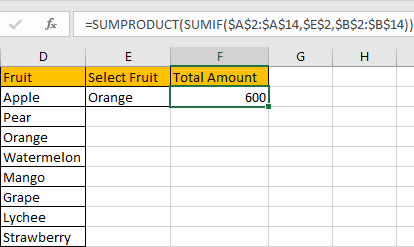Step 13: Select another fruit from dropdown menu. Verify that total amount is recalculated and updated properly.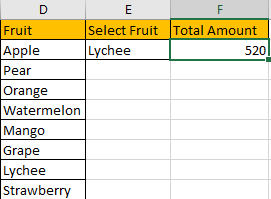### Related Functions

• Excel SUMPRODUCT function
The Excel SUMPRODUCT function multiplies corresponding components in the given one or more arrays or ranges, and returns the sum of those products.The syntax of the SUMPRODUCT function is as below:= SUMPRODUCT (array1,[array2],…)…
• Excel SUMIF Function
The Excel SUMIF function sum the numbers in the range of cells that meet a single criteria that you specify. The syntax of the SUMIF function is as below:=SUMIF (range, criteria, [sum_range])…
Related Posts

Case Sensitive Lookup with SUMPRODUCT and EXACT

Today, we will show you how to use SUMPRODUCT and EXACT to perform a case sensitive exact match. In this article, we provide a simple example to calculate bonus for employees whose names are case-sensitive. If you meet similar scenarios ...

Average per Week by Formula in Excel

We usually apply AVERAGE function or relevant functions to return average directly in Excel worksheet. But in some situations, only applying average relevant functions cannot figure out our problem. Sometimes we can create a formula with functions and mathematical operation ...

How to Sum if Equal to Many Items or A Range in Excel

If we want to sum numbers from a range with criteria, we often select SUMIF of SUMIFS functions as the first choice to create a formula. The criteria can be a number or an array or a collection of some ...

How to Sum if Equal to X or Y in Excel

In daily work, if we want to sum numbers from a range, and only sum the numbers which being equal to X or Y in the range, we can create a formula with Excel build-in functions to get the result. ...

How To Sum the Largest N Values in Excel

Sometimes we may want to sum the largest N numbers or top N numbers in a range. In this article, we will show you the method of “SUM the Largest N Numbers” by a simple formula which consist of SUMPRODUCT ...

How to Sum for Cell Contains Formula Only in Excel

Sometimes values are created by formulas in cells. If we want to sum values which are created by formulas from a range, but some values which are hardcoded also list in the same range, how can we filter out matched ...

How to Sum the Smallest N Values in Excel

Sometimes we may want to sum the first smallest N numbers in a range in Excel. In this article, we will show you the method of “SUM the Smallest N Numbers” by a simple formula which consist of SUMPRODUCT and ...

How to Sum by SUMPRDUCT with One Specific Criteria Multiple Columns in Excel

Sometimes we may meet the case that to sum numbers based on one specific criteria. In this article, we will show you the method to resolve this problem by formula with the help of Excel SUMPRODUCT function. SUMPRODUCT can filter ...

How to Sum by SUMPRDUCT with Specific Criteria in Excel

Sometimes we may meet the case that to sum numbers based on two or more specific criteria. In this article, we will show you the method to resolve this problem by formulas with the help of Excel SUMPRODUCT function. SUMPRODUCT ...

How to Count Unique Numeric Values in a Range in Excel

This post will guide you how to count unique numeric values in the given range in Excel 2013/2016 or Excel office 365. How do I count the unique numeric values in a list of data with some duplicate values using ...

Sidebar